Realization of Logic Gate Using Universal gates

• Last Updated : 25 Nov, 2021

In Boolean Algebra, the NAND and NOR gates are called universal gates because any digital circuit can be implemented by using any one of these two i.e. any logic gate can be created using NAND or NOR gates only.

Every logic gate has a representation symbol. The below image shows a graphical representation of all logic gates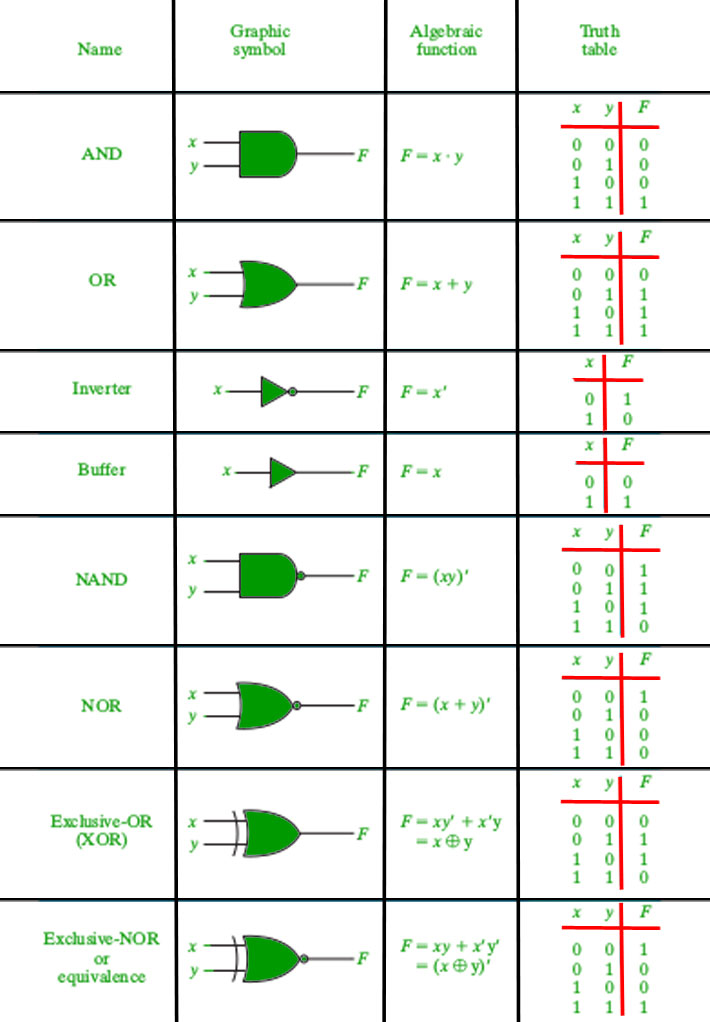Graphical representation of logic gates.

1. Implementation of AND Gate using Universal gates.

a) Using NAND Gates

The AND gate can be implemented by using two NAND gates in the below fashion: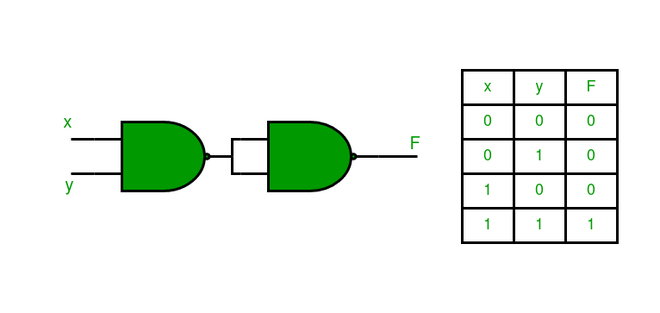b) Using NOR Gates

Implementation of AND gate using only NOR gates as shown below: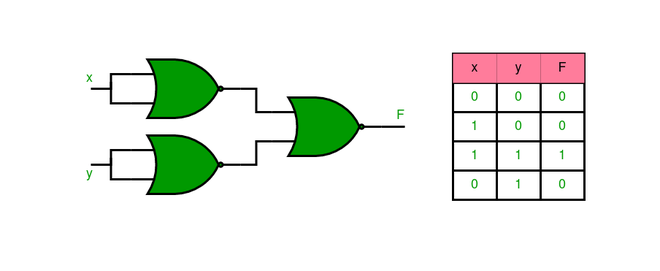2. Implementation of OR Gate using Universal gates.

a) Using NAND Gates

The OR gate can be implemented using the NAND gate as below: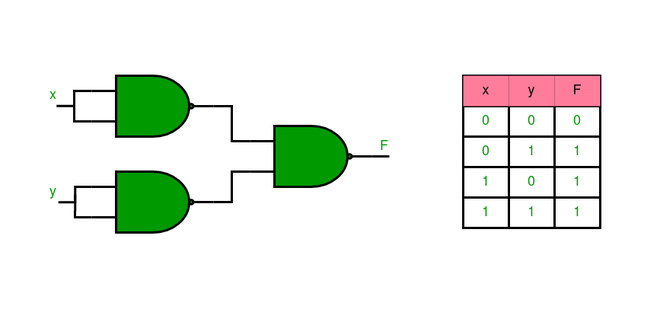b) Using NOR Gates

Implementation of OR gate using two NOR gates as shown in the picture below: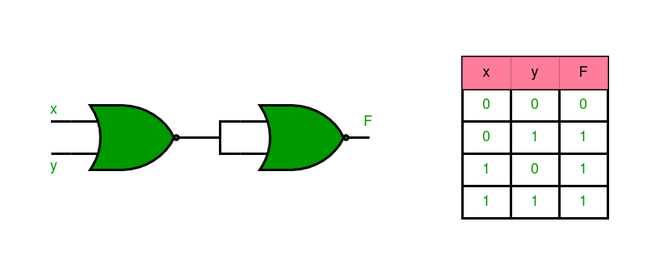3. Implementation of NOT Gate using Universal gates.

a) Using NAND Gates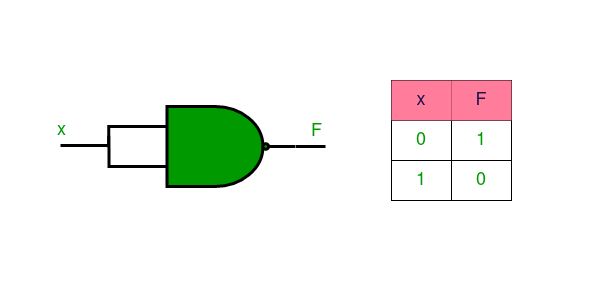b) Using NOR Gates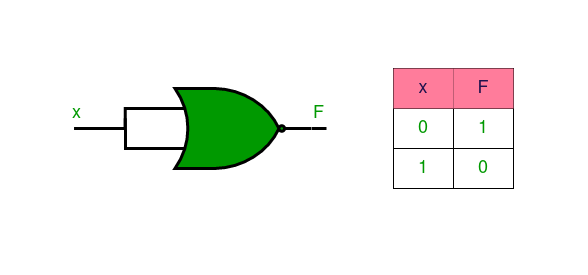4. Implementation of XOR Gate using Universal gates.

a) Using NAND Gates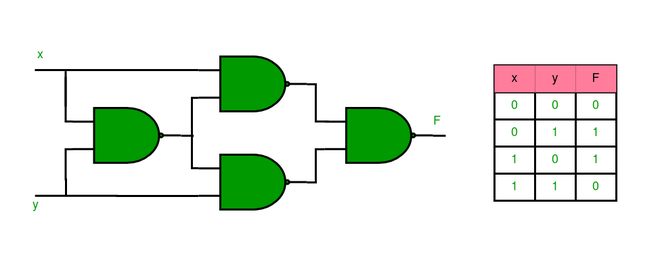b) Using NOR Gates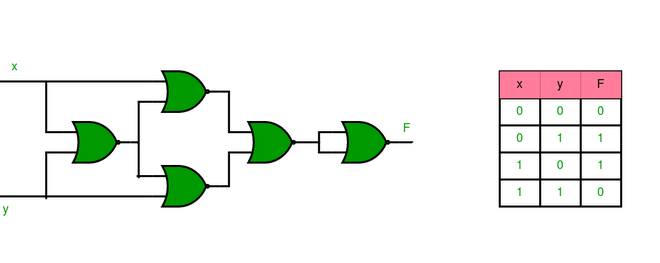5. Implementation of XNOR Gate using Universal gates.

a) Using NAND Gate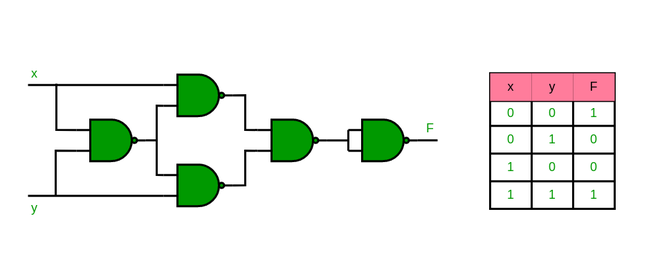b) Using NOR Gate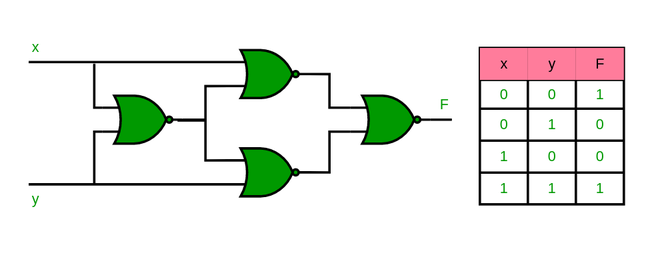6. Implementation of NOR Gate using NAND Gates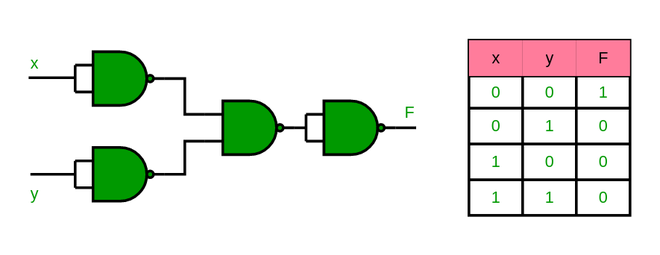7. Implementation of NAND Gate using NOR Gates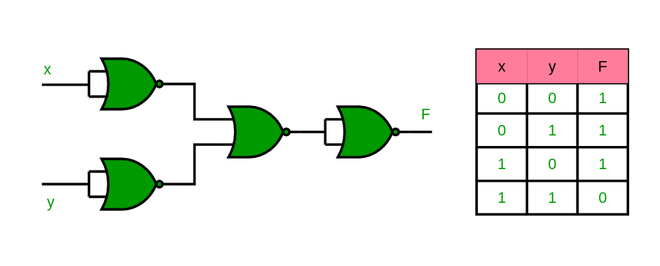My Personal Notes arrow_drop_up